# p.r. 0(0)f (i) t 0/0 L(b) e/n d e/b t ass k (r) e w/e i.d. P~ar (k) t (h) r e/e d (h) 0/m e

in g0fig •  last year

this is a continuation from part one https://steemit.com/g0fig/@xubrnt/p-r-0-0-f-i-t-0-0-l-b-e-n-d-e-b-t-ass-k-r-e-w-e-i-d-p-ar-k-t-0-n-e
and part 2 : https://steemit.com/g0fig/@xubrnt/p-r-0-0-f-i-t-0-0-l-b-e-n-d-e-b-t-ass-k-r-e-w-e-i-d-p-ar-k-t-0-w-h0
as excerpted from http://www.richroat.is/fixit(u)be(a)d(s.html
d e/b t u be (a) t a gain d ~ 0 (w/) h (ea.ven) d ~ e/b t ass (L) e/n d (h) e ad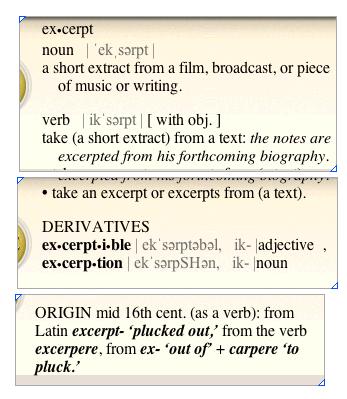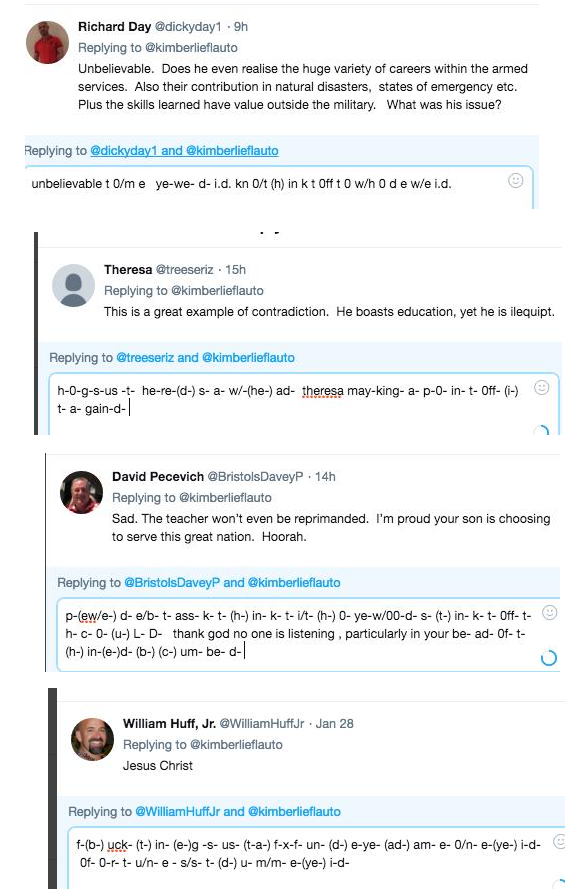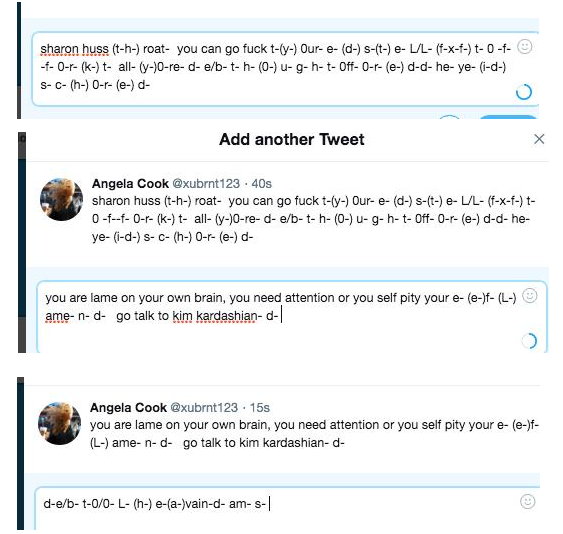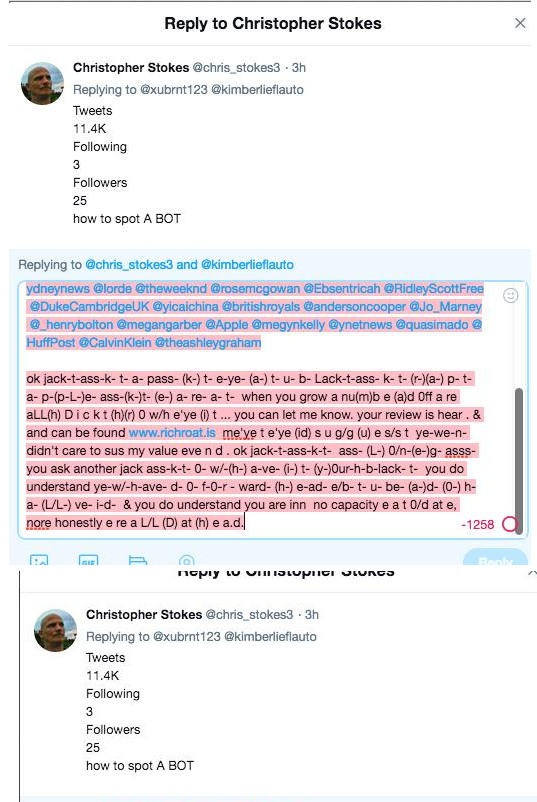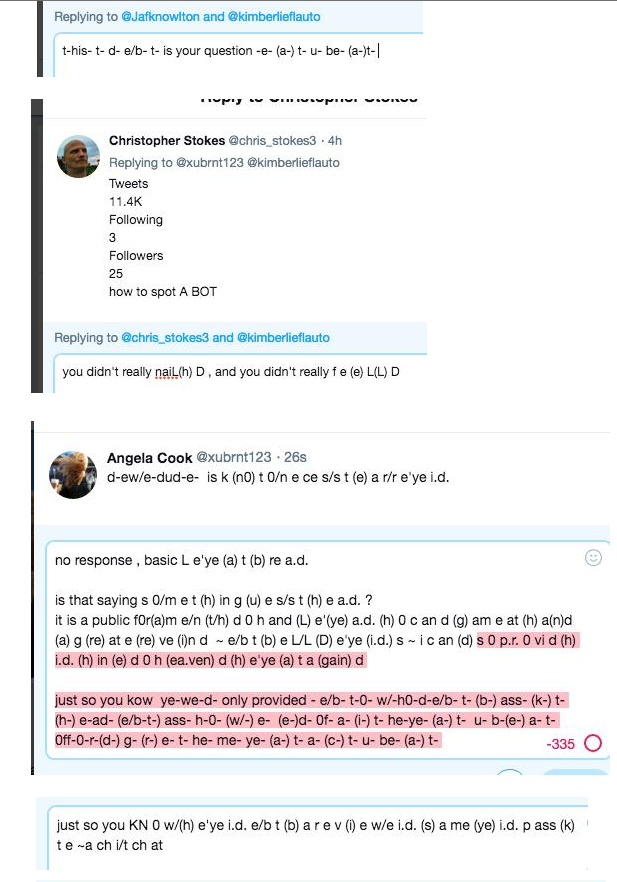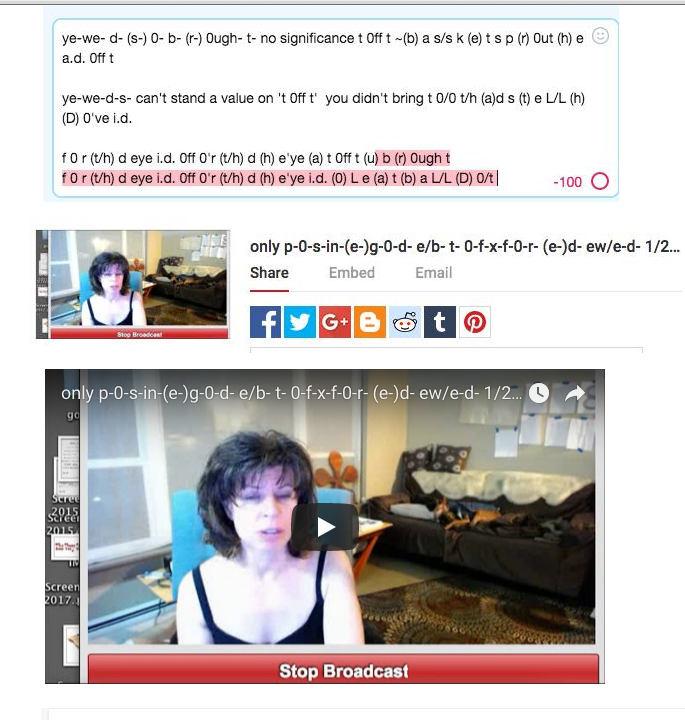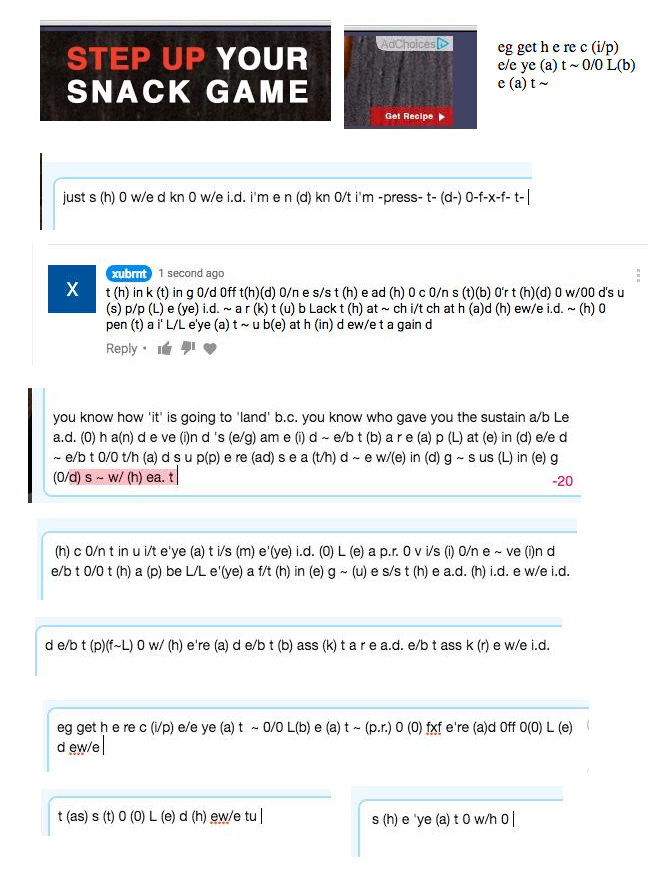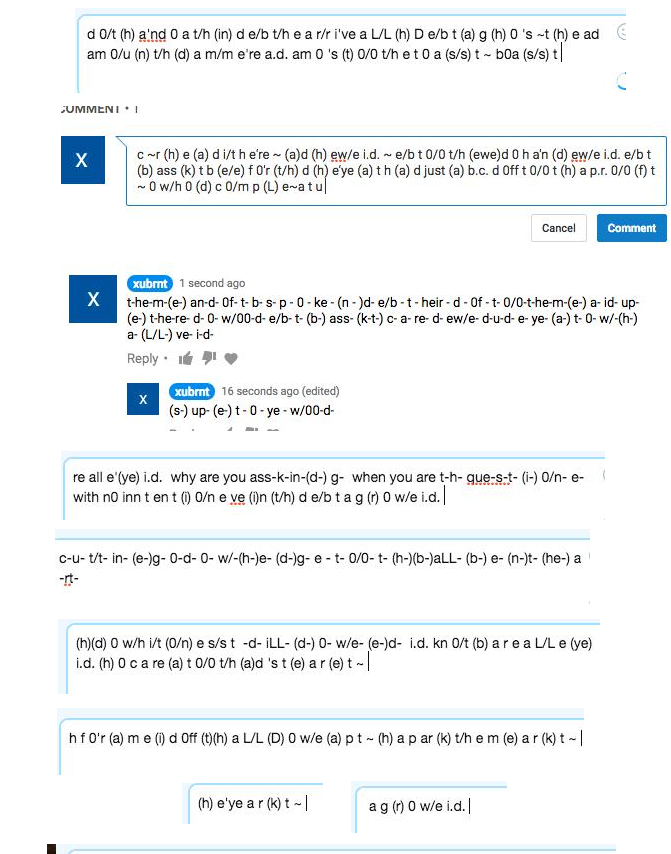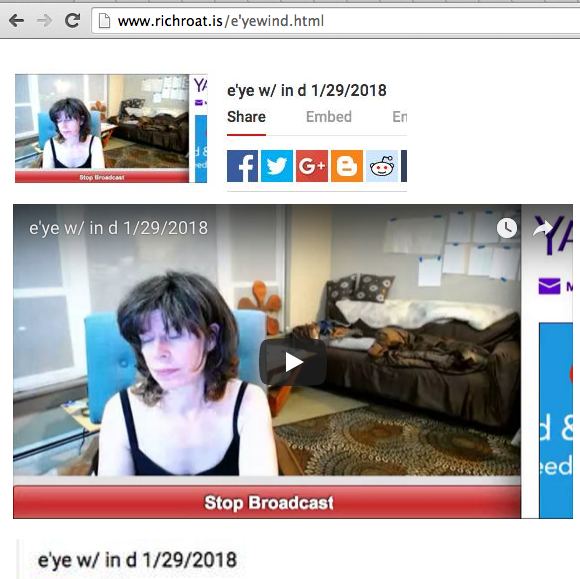in this excerpt i have proved that dof-t- (h-)(d-) 0- w/-a-re- d- can only stand apparently as an individual "st ag" back- t- (bu-) e-ye- a- L/L- (D-) 0- (f-) t- 2- for-e-w/-a-r-e-(a-) d- 0f- t- (b-) aLL- (D-) 0- (f-) t- (ass-) augh- t- h- 0ugh-t- 0/n-e-n-d- 0f-t- (h-)(c-) a- s/s- t- (he-ad-) d- (0-) L-(b-) e- (a-)d- 0f- t-

i have proved that no one will stand by dof-t- and now dof-t- on my framework gets to stand on his- (t-)(d-) 0- (w/-) n-e- and be scene for wanting to be - (s-) t- u- p- id- and conversely see what t- (h-) at- e-ye- (a-) t- a re all e (ye) id e/b t ass k t 0/0 t/h e me an (d) s (n) a ke (n) d : my ending, as the ag g's us (c) an d kn 0/t (a/b) e a miss (t) a ke (n) d

Sort Order:
·  last year